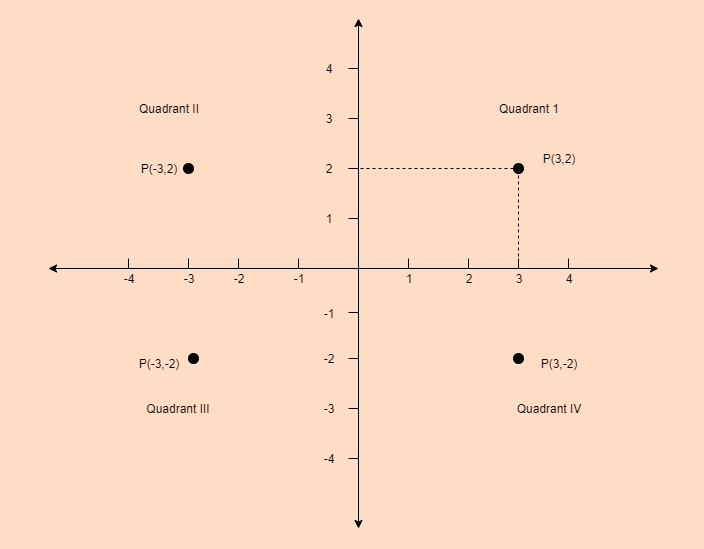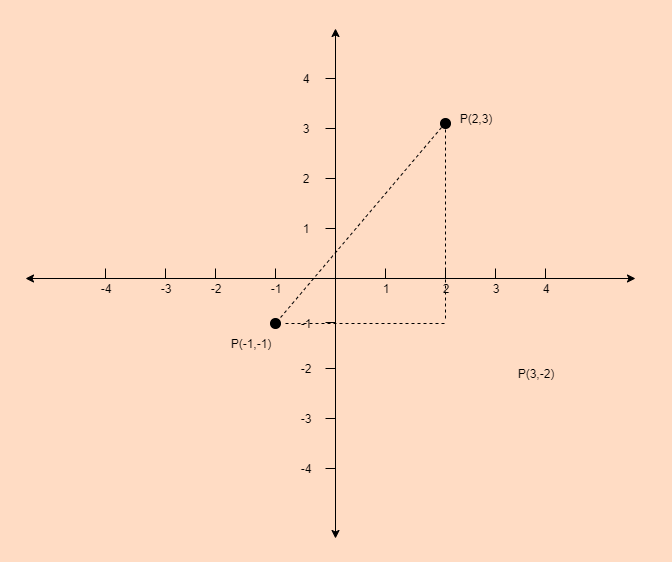GFG App
Open AppBrowser
Continue

# GRE Algebra | Coordinate Geometry

In the coordinate geometry, all the points are located on the coordinate plane. Coordinate plane is also called xy-plane.

The concepts of xy-plane are:

• The horizontal number line is called the x-axis and vertical number line is called the y-axis.
• The intersection point of both the axes is called the origin, denoted by O.
• The positive half of the x-axis right of the origin and negative half of x-axis is left to the origin.
• The positive half of the y-axis is above the origin and negative half of y-axis is below the origin.
• Two axes divide the plane into four Quadrants I, II, III and IV starting.In xy-plane every point is denoted by P(x, y). Here first number is called the x-coordinate and second number is called the y-coordinate. A point having coordinates (3, 2) is located 3 units to the right of y-axis and 2 units above to the x-axis as shown in the above plane.

Distance between two points:-
In xy-plane the distance between the two points (x1, y1) and (x2, y2) can be found by using the Pythagoras theorem.

Example: Find the distance between the points (2, 3) and (-1, -1).

Explanation:Distance between two points can be calculated using the below formula:

`Distance = √ [ (x2 - x1)2 + (y2 - y1)2) ] `

Put all the values,

```Distance
=  √[ (-1 - 2)2 + (-1 - 3)2) ]
=  √[ (-3)2 + (-4)2) ]
= √[ 9 + 16 ]
= √25
= 5 ```

Hence, distance between these two points is 5 unit.

My Personal Notes arrow_drop_up